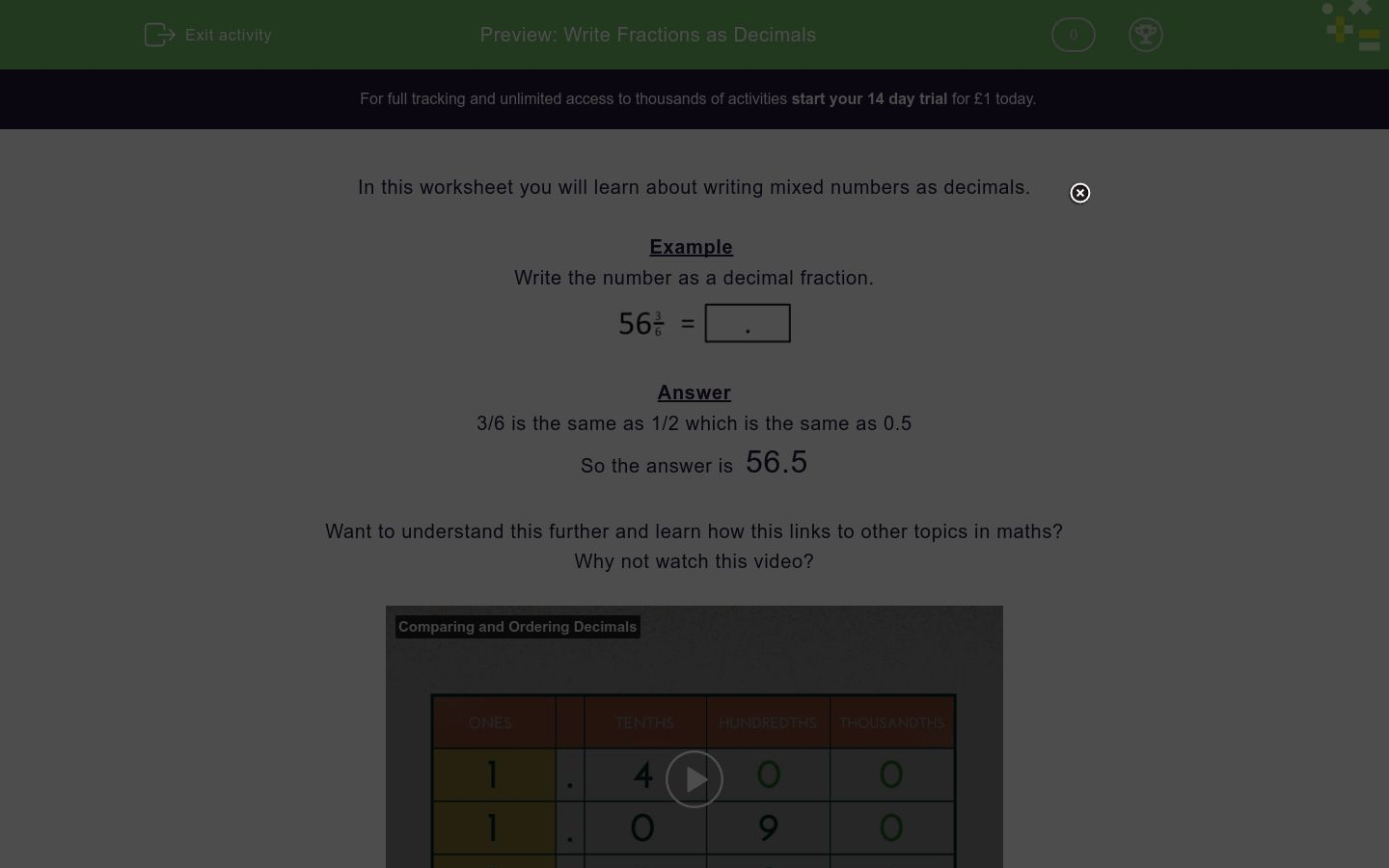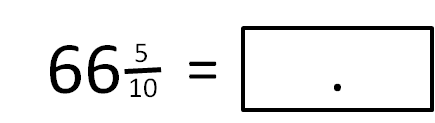# Write Fractions as Decimals

In this worksheet, students understand how to write a fraction as a decimal number.Key stage:  KS 2

Curriculum topic:   Number: Fractions and Decimals

Curriculum subtopic:   Write Decimal Equivalents

Difficulty level:### QUESTION 1 of 10

In this worksheet you will learn about writing mixed numbers as decimals.

Example

Write the number as a decimal fraction.3/6 is the same as 1/2 which is the same as 0.5

Want to understand this further and learn how this links to other topics in maths?
Why not watch this video?

Write the number as a decimal fraction.Write the number as a decimal fraction.

43 and four eighths

Write the number as a decimal fraction.Write the number as a decimal fraction.

97 and five tenths

Write the number as decimal fraction.

43 and a half

Write the number as a decimal fraction.Write the number as a decimal fraction.

71½

Write the number as a decimal fraction.

85 and two quarters

Write the number as a decimal fraction.Write the number as a decimal fraction.

92½

• Question 1

Write the number as a decimal fraction.76.5
• Question 2

Write the number as a decimal fraction.

43 and four eighths

43.5
• Question 3

Write the number as a decimal fraction.35.8
• Question 4

Write the number as a decimal fraction.

97 and five tenths

97.5
• Question 5

Write the number as decimal fraction.

43 and a half

43.5
• Question 6

Write the number as a decimal fraction.66.5
• Question 7

Write the number as a decimal fraction.

71½

71.5
• Question 8

Write the number as a decimal fraction.

85 and two quarters

85.5
• Question 9

Write the number as a decimal fraction.78.2
• Question 10

Write the number as a decimal fraction.

92½

92.5
---- OR ----

Sign up for a £1 trial so you can track and measure your child's progress on this activity.

### What is EdPlace?

We're your National Curriculum aligned online education content provider helping each child succeed in English, maths and science from year 1 to GCSE. With an EdPlace account you’ll be able to track and measure progress, helping each child achieve their best. We build confidence and attainment by personalising each child’s learning at a level that suits them.

Get started